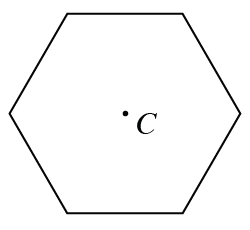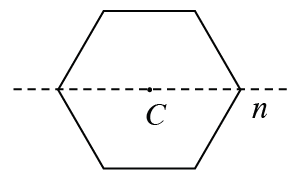### Home > GC > Chapter 1 > Lesson 1.2.4 > Problem1-86

1-86.At right is a diagram of a regular hexagon with center $C$. A polygon is regular if all sides are equal and all angles are equal. Copy this figure on your paper, then answer the questions below.

1. Draw the result of rotating the hexagon about its center $180º$. Explain what happened. When this happens, the shape has rotation symmetry.

The orientation of the hexagon does not change.

2.What is the result when the original hexagon is reflected across line $n$, as shown at right? A shape with this quality is said to have reflection symmetry and line $n$ is a line of symmetry of the hexagon (not of the reflection).

The orientation of the hexagon does not change.

3. Does a regular hexagon have any other lines of symmetry? That is, are there any other lines you could fold across so that both halves of the hexagon will match up? Find as many as you can.